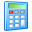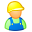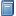Widgets# Labor Cost Calculator

Determine the impact of labor on total production costs and part costs. Labor costs are affected by the production time, labor rate, number of workers, and labor usage. Using these parameters, the total labor cost for production will be calculated, as well as the cost that can be attributed to each part.

## Labor Cost Calculator

Order quantity: \$ \$